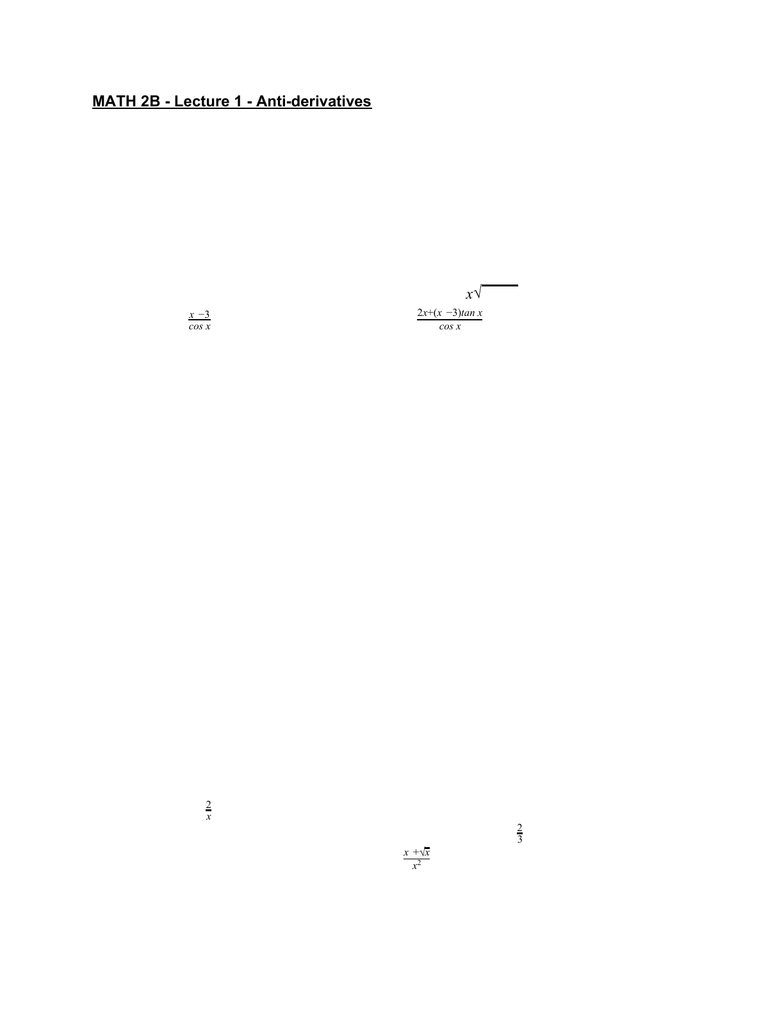Class Notes (1,100,000)
US (460,000)
UC-Irvine (10,000)
MATH (1,000)
MATH 2B (600)
Lecture 1

# MATH 2B Lecture Notes - Lecture 1: Antiderivative, Mean Value TheoremPremium

Department
Mathematics
Course Code
MATH 2B
Professor
ERJAEE, G.
Lecture
1

This preview shows half of the first page. to view the full 3 pages of the document.MATH 2B - Lecture 1 - Anti-derivatives
Anti-differentiation is exactly what it sounds like: the opposite of differentiation. That is,
given a function f, can we nd a function F whose derivative is f.
Denition. An anti-derivative of a function f is a function F such that F’(x) = f(x) for all x.
Examples
1. is an anti-derivative of (x) sin xF = (x)os xf =c
2. is an anti-derivative of (x)x)F= ( 2+ 3 3/2 (x) 3xf = x2+ 3
3. is an anti-derivative of (x)F=x3
2
cos x(x)f=cos x
2x+(x3)tan x
2
.
We can easily check the veracity of these statements by differentiating F(x). But what if
you are asked to nd an anti-derivative, not just check something you’ve been given? In
general this is a hard problem.
Differentiation is often described as easy in the sense that nice functions can
usually be differentiated via familiar rules
Anti-differentiation is hard: very few functions have anti-derivatives that can
easily be computed
○ Example
: It is easy to find the derivative of , but can you find an(x)f=ex2
anti-derivative of f(x)?
The only method that really exists for explicitly computing anti-derivatives is guess and
differentiate! Every famous rule that you’ll study in Integration (substitution, integration
by parts, etc.) is merely the result of guessing a general anti-derivative and checking
Anti-derivatives of Common Functions
Guessing is, of course, easier if you have familiarity with differentiation. With
each of the following functions f(x) you should be able to guess the chosen
anti-derivative F(x) just from what you know about derivatives
Examples
1. has an anti-derivative of (x)f= 3 + x
2(x)x ln|x|f= 3 + 2
2. has an anti-derivative (x)sec x ef = 3 2− 2 3x(x)tan x ef = 3 3
23x
3. Find an anti-derivative of (x)os xg =c+x2
x+
3x